## Introduction to Inequalities

Inequalities are used to demonstrate relationships between numbers or expressions.

### Learning Objectives

Explain what inequalities represent and how they are used

### Key Takeaways

#### Key Points

• An inequality describes a relationship between two different values.
• The notation $a < b$ means that $a$ is strictly smaller in size than $b$, while the notation $a > b$ means that $a$ is strictly greater than $b$.
• The notion $a \leq b$ means that $a$ is less than or equal to $b$, while the notation $a \geq b$ means that $a$ is greater than or equal to $b$.
• Inequalities are particularly useful for solving problems involving minimum or maximum possible values.

#### Key Terms

• number line: A visual representation of the set of real numbers as a series of points.
• inequality: A statement that of two quantities one is specifically less than or greater than another.

In mathematics, inequalities are used to compare the relative size of values. They can be used to compare integers, variables, and various other algebraic expressions. A description of different types of inequalities follows.

### Strict Inequalities

A strict inequality is a relation that holds between two values when they are different. In the same way that equations use an equals sign, =, to show that two values are equal, inequalities use signs to show that two values are not equal and to describe their relationship. The strict inequality symbols are $<$ and $>$.

Strict inequalities differ from the notation $a \neq b$, which means that a is not equal to $b$. The $\neq$ symbol does not say that one value is greater than the other or even that they can be compared in size.

In the two types of strict inequalities, $a$ is not equal to $b$. To compare the size of the values, there are two types of relations:

1. The notation $a < b$ means that $a$ is less than $b$.
2. The notation $a > b$ means that $a$ is greater than $b$.

The meaning of these symbols can be easily remembered by noting that the “bigger” side of the inequality symbol (the open side) faces the larger number. The “smaller” side of the symbol (the point) faces the smaller number.

The above relations can be demonstrated on a number line. Recall that the values on a number line increase as you move to the right. The following therefore represents the relation $a$ is less than $b$:### $a < b$

$a$ is to the left of $b$ on this number line.

and the following demonstrates $a$ being greater than $b$:### $a > b$

$a$ is to the right of $b$ on this number line.

In general, note that:

• $a < b$ is equivalent to $b > a$; for example, $7 < 11$ is equivalent to $11> 7$.
• $a > b$ is equivalent to $b < a$; for example, $6 < 9$ is equivalent to $9 > 6$.

### Other Inequalities

In contrast to strict inequalities, there are two types of inequality relations that are not strict:

• The notation $a \leq b$ means that $a$ is less than or equal to $b$ (or, equivalently, “at most” $b$).
• The notation $a \geq b$ means that $a$ is greater than or equal to $b$ (or, equivalently, “at least” $b$).

### Inequalities with Variables

In addition to showing relationships between integers, inequalities can be used to show relationships between variables and integers.

For example, consider $x > 5$. This would be read as “$x$ is greater than 5″ and indicates that the unknown variable $x$ could be any value greater than 5, though not 5 itself. For a visualization of this, see the number line below: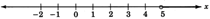### $x > 5$

Note that the circle above the number 5 is open, indicating that 5 is not included in possible values of $x$.

For another example, consider $x \leq 3$. This would be read as “$x$ is less than or equal to 3″ and indicates that the unknown variable $x$ could be 3 or any value less than 3. For a visualization of this, see the number line below: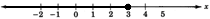### $x \leq 3$

Note that the circle above the number 3 is filled, indicating that 3 is included in possible values of $x$.

Inequalities are demonstrated by coloring in an arrow over the appropriate range of the number line to indicate the possible values of $x$. Note that an open circle is used if the inequality is strict (i.e., for inequalities using $>$ or $<$), and a filled circle is used if the inequality is not strict (i.e., for inequalities using $\geq$ or $\leq$).

### Solving Problems with Inequalities

Recall that equations can be used to demonstrate the equality of math expressions involving various operations (for example: $x + 5 = 9$). Likewise, inequalities can be used to demonstrate relationships between different expressions.

For example, consider the following inequalities:

• $x - 7 > 12$
• $2x + 4 \leq 25$
• $2x < y - 3$

Each of these represents the relationship between two different expressions.

One useful application of inequalities such as these is in problems that involve maximum or minimum values.

### Example 1

Jared has a boat with a maximum weight limit of 2,500 pounds. He wants to take as many of his friends as possible onto the boat, and he guesses that he and his friends weigh an average of 160 pounds. How many people can ride his boat at once?

This problem can be modeled with the following inequality:

$160n \leq 2500$

where $n$ is the number of people Jared can take on the boat. To see why this is so, consider the left side of the inequality. It represents the total weight of $n$ people weighing 160 pounds each. The inequality states that the total weight of Jared and his friends should be less than or equal to the maximum weight of 2,500, which is the boat’s weight limit.

There are steps that can be followed to solve an inequality such as this one. For now, it is important simply to understand the meaning of such statements and cases in which they might be applicable.

## Rules for Solving Inequalities

Arithmetic operations can be used to solve inequalities for all possible values of a variable.

### Learning Objectives

Solve inequalities using the rules for operating on them

### Key Takeaways

#### Key Points

• When you’re performing algebraic operations on inequalities, it is important to perform the same operation on both sides in order to preserve the truth of the statement.
• If both sides of an inequality are multiplied or divided by the same positive value, the resulting inequality is true.
• If both sides are multiplied or divided by the same negative value, the direction of the inequality changes.
• Inequalities involving variables can be solved to yield all possible values of the variable that make the statement true.

#### Key Terms

• inequality: A statement that of two quantities one is specifically less than or greater than another.

### Operations on Inequalities

When you’re performing algebraic operations on inequalities, it is important to conduct precisely the same operation on both sides in order to preserve the truth of the statement.

Each arithmetic operation follows specific rules:

Any value $c$ may be added to or subtracted from both sides of an inequality. That is to say, for any real numbers $a$, $b$, and $c$:

• If $a \leq b$, then $a + c \leq b + c$ and $a - c \leq b - c$.
• If $a \geq b$, then $a + c \geq b + c$ and $a - c \geq b - c$.

As long as the same value is added or subtracted from both sides, the resulting inequality remains true.

For example, consider the following inequality:

$12 < 15$

Let’s apply the rules outlined above by subtracting 3 from both sides:

\begin{align} 12 - 3 &< 15 - 3 \\ 9 &< 12 \end{align}

This statement is still true.

### Multiplication and Division

The properties that deal with multiplication and division state that, for any real numbers, $a$, $b$, and non-zero $c$:

If $c$ is positive, then multiplying or dividing by $c$ does not change the inequality:

• If $a \geq b$ and $c >0$, then $ac \geq bc$ and $\dfrac{a}{c} \geq \dfrac{b}{c}$.
• If $a \leq b$ and $c > 0$, then $ac \leq bc$ and $\dfrac{a}{c} \leq \dfrac{b}{c}$.

If $c$ is negative, then multiplying or dividing by $c$ inverts the inequality:

• If $a \geq b$ and $c <0$, then $ac \leq bc$ and $\dfrac{a}{c} \leq \dfrac{b}{c}$.
• If $a \leq b$ and $c < 0$, then $ac \geq bc$ and $\dfrac{a}{c} \geq \dfrac{b}{c}$.

Take note that multiplying or dividing an inequality by a negative number changes the direction of the inequality. In other words, a greater-than symbol becomes a less-than symbol, and vice versa.

To see these rules applied, consider the following inequality:

$5 > -3$

Multiplying both sides by 3 yields:

\begin{align} 5 (3) &> -3 (3) \\ 15 &> -9 \end{align}

We see that this is a true statement, because 15 is greater than 9.

Now, multiply the same inequality by -3 (remember to change the direction of the symbol because we’re multiplying by a negative number):

\begin{align} 5 (-3) &< -3 (-3) \\ -15 &< 9 \end{align}

This statement also holds true. This demonstrates how crucial it is to change the direction of the greater-than or less-than symbol when multiplying or dividing by a negative number.

### Solving Inequalities

Solving an inequality that includes a variable gives all of the possible values that the variable can take that make the inequality true. To solve an inequality means to transform it such that a variable is on one side of the symbol and a number or expression on the other side. Often, multiple operations are often required to transform an inequality in this way.

To see how the rules of addition and subtraction apply to solving inequalities, consider the following:

$x - 8 \leq 17$

First, isolate $x$:

\begin{align} x - 8 + 8 &\leq 17 + 8 \\ x &\leq 25 \end{align}

Therefore, $x \leq 25$ is the solution of $x - 8 \leq 17$. In other words, $x - 8 \leq 17$ is true for any value of $x$ that is less than or equal to 25.

### Multiplication and Division

To see how the rules for multiplication and division apply, consider the following inequality:

$2x > 8$

Dividing both sides by 2 yields:

\begin{align} \dfrac{2x}{2} &> \dfrac{8}{2} \\ x &> \dfrac{8}{2} \\ x &> 4 \end{align}

The statement $x > 4$ is therefore the solution to $2x > 8$. In other words, $2x > 8$ is true for any value of $x$ greater than 4.

Now, consider another inequality:

$-\dfrac{y}{3} \leq 7$

Because of the negative sign involved, we must multiply by a negative number to solve for $y$. This means that we must also change the direction of the symbol:

\begin{align} \displaystyle -3 \left( -\frac{y}{3} \right) &\geq -3 (7)\\ y &\geq -3 (7) \\ y &\geq -21 \end{align}

Therefore, the solution to $-\frac{y}{3} \leq 7$ is $y \geq -21$. The given statement is therefore true for any value of $y$ greater than or equal to $-21$.

### Example

Solve the following inequality:

$3y - 17 \geq 19$

First, add 17 to both sides:

\begin{align} 3y - 17 + 17 &\geq 19 + 17 \\ 3y &\geq 36 \end{align}

Next, divide both sides by 3:

\begin{align} \dfrac{3y}{3} &\geq \dfrac{36}{3} \\ y &\geq \dfrac{36}{3} \\ y &\geq 12 \end{align}

### Special Considerations

Note that it would become problematic if we tried to multiply or divide both sides of an inequality by an unknown variable. If any variable $x$ is unknown, we cannot identify whether it has a positive or negative value. Because the rules for multiplying or dividing positive and negative numbers differ, we cannot follow this same rule when multiplying or dividing inequalities by variables. Variables can, however, be added or subtracted from both sides of an inequality.

## Compound Inequalities

A compound inequality involves three expressions, not two, but can also be solved to find the possible values for a variable.

### Learning Objectives

Solve a compound inequality by balancing all three components of the inequality

### Key Takeaways

#### Key Points

• A compound inequality is of the following form: $a < x < b$.
• There are two statements in a compound inequality. The first statement is $a < x$. The next statement is $x < b$. When we read this statement, we say “$a$ is less than $x$, and $x$ is less than $b$.”
• An example of a compound inequality is: $4 < x < 9$. In other words, $x$ is some number strictly between 4 and 9.
• A compound inequality may contain an expression, such as $1 < x - 6 < 8$; such inequalities can be solved for all possible values of $x$.

#### Key Terms

• compound inequality: An inequality that is made up of two other inequalities, in the form $a < x < b$.
• inequality: A statement that of two quantities one is specifically less than or greater than another.

#### Defining Compound Inequalities

A compound inequality is of the following form:

$a < x < b$

There are actually two statements here. The first statement is $a < x$. The next statement is $x < b$. This statement is therefore read as “$a$ is less than $x$, and $x$ is less than $b$.”

The compound inequality $a < x < b$ indicates “betweenness”—the number $x$ is between the numbers $a$ and $b$. Without changing the meaning, the statement $a<x$ can also be read as $x>a$. Therefore, the form $a < x < b$ can also be read as “$x$ is greater than $a$, and at the same time is less than $b$.”

Consider $4 < x < 9$. This states that $x$ is some number strictly between 4 and 9. For a visualization of this inequality, refer to the number line below. The numbers 4 and 9 are not included, so we place open circles on these points.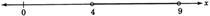### $4 < x < 9$

The above inequality on the number line.

Similarly, consider $-2 < z < 0$. In this case, $z$ is some number strictly between -2 and 0. Again, because the numbers -2 and 0 are not included, we place open circles on those points.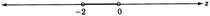### $-2 < x < 0$

The above inequality on the number line.

### Solving Compound Inequalities

Now consider $1 < x + 6 < 8$. The expression $x + 6$ represents some number strictly between 1 and 8. However, the meaning of this is difficult to visualize—what does it mean to say that an expression, rather than a number, lies between two points? Not to worry—we can still find all possible values of not only the expression, but the variable $x$ itself.

The statement $1 < x + 6 < 8$ says that the quantity $x + 6$ is between 1 and 8, a statement that will be true for only certain values of $x$.

To solve for possible values of $x$, we need to get $x$ by itself:

$1 - 6 < x + 6 - 6 < 8 - 6$

$-5 < x < 2$

Therefore, we find that if $x$ is any number strictly between -5 and 2, the statement $1 < x + 6 < 8$ will be true.

### Example 1

Solve $-3 < \dfrac{-2x-7}{5} < 7$.

Multiply each part to remove the denominator from the middle expression:

$-3\cdot (5) < \dfrac{-2x-7}{5} \cdot (5) < 7 \cdot (5)$

$-15 < -2x-7 < 35$

Isolate $x$ in the middle of the inequality:

$- 15 + 7 < -2x -7 + 7 < 35 + 7$

$- 8 < -2x < 42$

Now divide each part by -2 (and remember to change the direction of the inequality symbol!):

$\displaystyle \frac{-8}{-2} > \frac{-2x}{-2} > \frac{42}{-2}$

$4 > x > -21$

Finally, it is customary (though not necessary) to write the inequality so that the inequality arrows point to the left (i.e., so that the numbers proceed from smallest to largest):

$-21 < x < 4$

## Inequalities with Absolute Value

Inequalities with absolute values can be solved by thinking about absolute value as a number’s distance from 0 on the number line.

### Learning Objectives

Solve inequalities with absolute value

### Key Takeaways

#### Key Points

• Problems involving absolute values and inequalities can be approached in at least two ways: through trial and error, or by thinking of absolute value as representing distance from 0 and then finding the values that satisfy that condition.
• When solving inequalities that involve an an absolute value within a larger expression (for example, $\left|2x\right|+ 3>8$), it is necessary to algebraically isolate the absolute value and then algebraically solve for the variable.

#### Key Terms

• absolute value: The magnitude of a real number without regard to its sign; formally, -1 times a number if the number is negative, and a number unmodified if it is zero or positive.
• inequality: A statement that of two quantities one is specifically less than or greater than another.
• number line: A line that graphically represents the real numbers as a series of points whose distance from an origin is proportional to their value.

Consider the following inequality that includes an absolute value:

$|x| < 10$

Knowing that the solution to $\left|x\right|=10$ is $x = ± 10$, many students answer this question $x < ± 10$. However, this is wrong.

Here are two different, but both perfectly correct, ways to look at this problem.

### Trial and Error

What numbers work? That is to say, for what numbers is $\left|x\right| < 10$ a true statement? Let’s test some out.

4 works. -4 does too. 13 doesn’t work. How about -13? No: If $x = -13$, then $\left|x\right| = 13$, which is not less than 10.

By playing with numbers in this way, you should be able to convince yourself that the numbers that work must be somewhere between -10 and 10. This is one way to approach finding the answer.

### Absolute Value as Distance

The other way is to think of absolute value as representing distance from 0. $\left|5\right|$and $\left|-5\right|$ are both 5 because both numbers are 5 away from 0.

In this case, $\left|x\right| < 10$ means “the distance between $x$ and 0 is less than 10.” In other words, you are within 10 units of zero in either direction. Once again, we conclude that the answer must be between -10 and 10.

This answer can be visualized on the number line as shown below, in which all numbers whose absolute value is less than 10 are highlighted.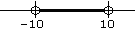Solution to $\left|x\right| < 10$: All numbers whose absolute value is less than 10.

It is not necessary to use both of these methods; use whichever method is easier for you to understand.

### Solving Inequalities with Absolute Value

More complicated absolute value problems should be approached in the same way as equations with absolute values: algebraically isolate the absolute value, and then algebraically solve for $x$.

For example, consider the following inequality:

$\left|2x\right| + 3>8$

It is difficult to immediately visualize the meaning of this absolute value, let alone the value of $x$ itself. It is necessary to first isolate the inequality:

\begin{align} \left|2x\right| + 3 - 3 &> 8 - 3 \\ \left|2x\right| &> 8 \end{align}

Now think about the number line. In those terms, this statement means that the expression $2x$ must be more than 8 places away from 0. Therefore, it must be either greater than 8 or less than -8. Expressing this with inequalities, we have:

$2x>8$ or $2x < -8$

We now have 2 separate inequalities. If each one is separately solved for $x$, we will see the full range of possible values of $x$. Consider them independently. First:

\begin{align} 2x &>8 \\ \dfrac{2x}{2} &> \dfrac{8}{2} \\ x &> 4 \end{align}

Second:

\begin{align} 2x &< -8 \\ \dfrac{2x}{2} &< \dfrac{-8}{2} \\ x &< -4 \end{align}

We now have two ranges of solutions to the original absolute value inequality:

$x > 4$ and $x < -4$

This can also be visually displayed on a number line: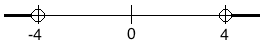Solution to $\left|2x\right| + 3>8$: The solution is any value of $x$ less than -4 or greater than 4.

### Example

Solve the following inequality:

$\left|x-2\right| + 10 > 7$

First, algebraically isolate the absolute value:

\begin{align} \left|x-2\right| + 10 - 10 &> 7 - 10 \\ \left|x-2\right| &> - 3 \end{align}

Now think: the absolute value of the expression is greater than –3. What could the expression be equal to? 2 works. –2 also works. And 0. And 7. And –10. Absolute values are always positive, so the absolute value of anything is greater than –3! All numbers therefore work.